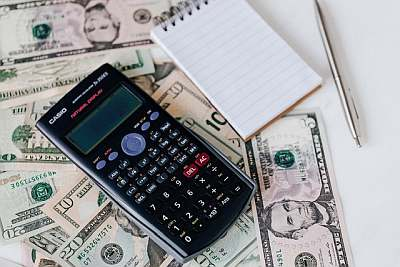# Effective Annual Rate Calculator

Instructions: Use this Effective Annual Rate Calculator to compute the effective annual rate (EAR) by indicating the yearly the interest rate ($$r$$) and the type of compounding (yearly, bi-yearly, quarterly, monthly, weekly, daily or continuously):Yearly Interest Rate $$(r)$$ =
Compounding Period:

## Effective Annual Rate Calculator

More about the this EAR calculator so you can better use this solver: The effective annual rate ($$EAR$$) corresponds to the actual rate that is carried by a nominal annual rate ($$r$$). The difference between the nominal annual rate $$r$$ and the effective annual rate $$EAR$$ is due to the fact that for the $$EAR$$ there is a number of compounding periods.

### How do you calculate effective annual rate?

For discrete compounding, the following formula is used:

$EAR = \left( 1+\frac{r}{k}\right)^{ k} - 1$

For continuous compounding, we get that $$k \to \infty$$, in which case we need to use the following formula instead.

$EAR = e^{r} - 1$

### How to compute the effective annual rate excel?

For example, assume that the nominal rate is $$r = 10\%$$ and the compounding is done monthly, so the number of compounding periods is $$k = 12$$. The following formula needs to be used in Excel to get the effective annual rate: =FV(10%/12, 12, 0, -1)-1, which would yield a EAR of 10.47%.

### How do you calculate the effective interest rate on a loan?

This is the same way you would calculate effective interest rate on a loan: You would take your nominal rate of $$r$$, and the number of compounding periods is $$k = 12$$, and you would use the Excel formula =FV(r/k, k, 0, -1)-1 . Or you could use our calculator, which will provide you will all the steps in the calculator.### Example of the calculation of the annual rate

Question: Find the effective rate of a nominal rate of 4%, compounded monthly.

Solution:

This is the information we have been provided with:

• The annual rate is $$r = 0.04$$, and the compounding is done monthly.

Therefore, the effective annual rate (EAR) is computed using the following formula:

$\begin{array}{ccl} EAR & = & \displaystyle \left( 1+\frac{r}{k}\right)^{ k } - 1 \\\\ \\\\ & = & \displaystyle \left( 1+\frac{0.04}{12}\right)^{ 12 } - 1 \\\\ \\\\ & = & 0.0407 = 4.0742\%\end{array}$ which means that the effective annual rate (EAR) for an annual rate of $$r = 0.04$$, with monthly compounding is $$EAR =0.0407 = 4.0742\%$$.

### Other financial calculators you may be interested in

You may be interested in computing other financial rates, such as the yield to maturity , for example.